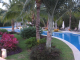# Grid Edit Mode: Selecting Item from KendoComboBox does not display selected value.

GridTop achievementsRank 1
KobusVisagie asked on 06 Jul 2012, 11:01 AM
Hello guys,

I have the following grid:

`_grid.kendoGrid({`
`    ``navigatable: ``true``,`
`    ``pageable: ``true``,`
`    ``editable: ``true``,`
`    ``toolbar: [``"create"``, ``"save"``],`
`    ``columns: (_columns.length > 0) ? _columns : ``null``,`
`    ``dataSource: {`
`        ``data: (_view.data > 0) ? _view.data : ``null``,`
`        ``pageSize: 100,`
`        ``schema: {`
`            ``model: {`
`                ``id: ``"id"``,`
`                ``fields: _spec`
`            ``}`
`        ``}`
`    ``},`
`});`

The columns for the grid, and the fields for the schema's model are generated as follows:

`var` `_filter = ``null``;`
`var` `_columns = ``new` `Array();`

`var` `_spec = ``new` `Array();`

`for` `(_field ``in` `_view.fields) {`
`    ``if` `(``typeof` `_view.fields[_field] == ``'undefined'``) ``continue``;`

`    ``var` `_f = _view.fields[_field];`

`    ``if` `(_f.type == ``'DropDown'``) {`
`        ``_filter = {`
`            ``field: _f.key,`
`            ``title: _f.title,`
`            ``editor: setEditor(_f)`
`        ``};`

`        ``_spec[_f.key] = _f.key;`
`    ``}`
`    ``else` `{`
`        ``_filter = {`
`            ``field: _f.key,`
`            ``title: _f.title`
`        ``};`

`        ``_spec[_f.key] = {`
`            ``type: getKendoFieldType(_f),`
`            ``//editable: true,`
`            ``validation: {`
`                ``required: ((_f.required) ? ``true` `: ``false``)`
`                ``//min: 1`
`            ``}`
`        ``};`
`    ``}`

`    ``_columns.push(_filter);`
`}`

`_command = {`
`    ``command: ``'destroy'``,`
`    ``title: ``' '``,`
`    ``width: 110`
`};`

`_columns.push(_command);`

When I click an item in the grid that's supposed to be a DropDown, it calls the method "setEditor" as per the code above, it looks like this:

`setEditor: ``function` `(_field) {`
`    ``var` `_context = ``this``;`

`    ``return` `function` `(container, options) {`
`        ``\$.post(``'/api/'``,`
`        ``{`
`            ``method: ``'GET'``,`
`            ``api: context.api + _field.populateapi,`
`            ``json: ``''`
`        ``},`
`        ``function` `(data) {`
`            ``if` `(!_context.valid(data, ``true``)) ``return``;`
`            ``var` `_data = \$.parseJSON(data);`

`            ``\$(``'<input data-bind="value:'` `+ options.field + ``'" />'``)`
`                ``.appendTo(container)`
`                ``.kendoComboBox({`
`                    ``autobind: ``false``,`
`                    ``dataValueField: ``'id'``,`
`                    ``dataTextField: ``'datatext'``,`
`                    ``dataSource: _data`
`                ``});`
`        ``});`
`    ``}`
`},`

The grid gets generated just fine. When I click on one of the fields that's supposed to be a drop-down field, it works fine too, the combo-box gets generated. When I selected something for the drop-down, it doesn't dissapear, it also doesn't put the selected text in the field on the grid. I looked at many examples, but I can't figure why this isn't working.

Any help would be appreciated. Thanks for your time!

## 3 Answers, 1 is accepted

0KobusVisagie
Top achievementsRank 1
answered on 09 Jul 2012, 06:19 AM
0KobusVisagie
Top achievementsRank 1
answered on 09 Jul 2012, 11:31 AM
I found a workaround to this problem. Thanks for the help!
0Jerry T.
Top achievementsRank 1
answered on 09 Jul 2012, 01:52 PM
Gregory,

I, too, have issues with tabbing out of a cell after selecting something from a dropdown only to have that cell's value changed to the ID value, not the Text value.

Jerry
Tags
GridKobusVisagie
Top achievementsRank 1Rank 1Rank 1